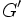# Finitely generated FZ-group

This page describes a group property obtained as a conjunction (AND) of two (or more) more fundamental group properties: finitely generated group and FZ-group
View other group property conjunctions OR view all group properties

## Definition

### Equivalent definitions in tabular format

No. Shorthand A group is termed a finitely generated FZ-group if ... A group$G$ is termed a finitely generated FZ-group if ...
1 it is finitely generated and FZ it is both a finitely generated group and a FZ-group.$G$ has a finite generating set and its inner automorphism group is a finite group.
2 FZ and center is finitely generated abelian it is a FZ-group and its center is a finitely generated abelian group.$G/Z(G)$ is a finite group and$Z(G)$ is a finitely generated abelian group.
3 finitely generated and finite derived subgroup it is a finitely generated group and also a group with finite derived subgroup, i.e., its derived subgroup is a finite group.$G$ has a finite generating set and the derived subgroup$G'$ is a finite group.
4 finitely generated and FC it is a finitely generated group and a FC-group$G$ has a finite generating set and every conjugacy class in$G$ is finite.

### Equivalence of definitions

Implication direction Proof
(1) implies (2) Schreier's lemma gives that any subgroup of finite index in a finitely generated group is finitely generated, hence, the center is finitely generated.
(2) implies (1) If the center is finitely generated, then so is the whole group, because the center has finite index, so we need to add in only finitely many elements to the generating set of the center to get a generating set of the whole group.
(1) implies (3) follows from FZ implies finite derived subgroup (also known as the Schur-Baer theorem).
(3) implies (4) follows from finite derived subgroup implies FC (straightforward).
(4) implies (1) see finitely generated and FC implies FZ.

## Relation with other properties

### Stronger properties

Property Meaning Proof of implication Proof of strictness (reverse implication failure) Intermediate notions
finite group has only finitely many elements (obvious) any infinite finitely generated abelian group provides a counterexample. |FULL LIST, MORE INFO
finitely generated abelian group finitely generated and abelian (obvious) any finite non-abelian group provides a counterexample. |FULL LIST, MORE INFO

### Weaker properties

Property Meaning Proof of implication Proof of strictness (reverse implication failure) Intermediate notions
FZ-group center has finite index (obvious) any abelian group that is not finitely generated gives a counterexample. |FULL LIST, MORE INFO
group with finite derived subgroup derived subgroup is finite (from definition) |FULL LIST, MORE INFO
BFC-group there is a common finite bound on the size of all conjugacy classes FZ-group|FULL LIST, MORE INFO